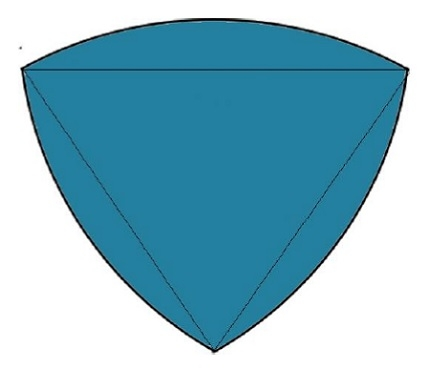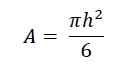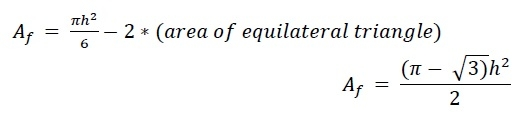# Area of Reuleaux Triangle?

Here we will see how to calculate the area of Reuleaux Triangle like below. The Reuleaux Triangle has one equilateral triangle inside it. Suppose its height is h, this shape is made by the intersection of three circles.There are three circular sectors. The area of each sector is −Since the area of the equilateral triangle is added three times, then we have to subtract them. So the final area is −## Example

#include <iostream>
#include <cmath>
using namespace std;
float areaReuleaux(float h) {
if (h < 0) //if h is negative it is invalid
return -1;
float area = ((3.1415 - sqrt(3)) * h * h)/2;
return area;
}
int main() {
float height = 6;
cout << "Area of Reuleaux Triangle: " << areaReuleaux(height);
}

## Output

Area of Reuleaux Triangle: 25.3701